# On algorithms for computing the sums of powers of arithmetic progressions

Peter J. Shiue, Shen C. Huang and Eric Jameson
Notes on Number Theory and Discrete Mathematics
Print ISSN 1310–5132, Online ISSN 2367–8275
Volume 26, 2020, Number 4, Pages 113—121
DOI: 10.7546/nntdm.2020.26.4.113-121

## Details

### Authors and affiliations

Peter J. ShiueDepartment of Mathematical Sciences, University of Nevada, Las Vegas,
Las Vegas, NV 89154-4020, USA

Shen C. HuangDepartment of Mathematical Sciences, University of Nevada, Las Vegas,
Las Vegas, NV 89154-4020, USA

Eric JamesonDepartment of Mathematical Sciences, University of Nevada, Las Vegas,
Las Vegas, NV 89154-4020, USA

### Abstract

This paper is concerned with sums of powers of arithmetic progressions of the form, where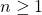,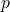is a non-negative integer, and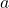and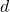are complex numbers with. This paper gives an elementary proof to a theorem presented by Laissaoui and Rahmani  as well as an algorithm based on their formula. Additionally, this paper presents a simplification to Laissaoui and Rahmani’s formula that is better suited to computation, and a second algorithm based on this simplification. Both formulas use Stirling numbers of the second kind, which are the number of ways to partitionlabelled objects into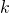nonempty unlabelled subsets . An analysis of both algorithms is presented to show the theoretical time complexities. Finally, this paper conducts experiments with varying values of. The experimental results show the proposed algorithm remains around 10% faster asincreases.

### Keywords

• Analysis of algorithms
• Arithmetic progressions
• Dynamic programming
• Stirling number of the second kind

• 05A10
• 11B25
• 11B65
• 11B73
• 68N15
• 68Q25

### References

1. Aigner, M. (2010). Discrete Mathematics. American Mathematical Society.
2. Bein, W. (2013). Advanced techniques for dynamic programming. In: Pardalos, P., M., Du, D.-Z., & Graham, R. L. (Editors), Handbook of Combinatorial Optimization, Chapter 2, Springer, pp. 41–92.
3. Bounebirat, F., Laissaoui, D., & Rahmani, M. (2018). Several explicit formulae of sums and hyper-sums of powers of integers. Online Journal of Analytic Combinatorics, 13.
4. Charalambides, C. A. (2002). Enumerative Combinatorics. Chapman & Hall / CRC Press, pp. 278–279.
5. Chen, W. Y. C., Fu, A. M., & Zhang, I. F. (2009). Faulhaber’s theorem on power sums. Discrete Mathematics, 309 (10), 2974–2981.
6. Howard, F.T. (1996). Sums of powers of integers via generating functions. Fibonacci Quarterly, 34 (3), 244–256.
7. Hsu, L. C., & Shiue, P. J.-S. (1999). On certain summation problems and generalizations of Eulerian polynomials and numbers. Discrete Mathematics, 204 (1-3), 237–247.
8. Knuth, D. E. (1993). Johann Faulhaber and sums of powers. Mathematics of Computation, 61 (203), 277–294.
9. Laissaoui, D., & Rahmani, R. (2017). An explicit formula for sums of powers of integers in terms of Stirling numbers. Journal of Integer Sequences, 20 (4), Aricle 17.4.8.
10. Pita-Ruiz, C. (2018). On a generalization of Eulerian numbers. Notes on Number Theory and Discrete Mathematics, 24 (1), 16–42.
11. Vassilev, P., & Vassilev-Missana, M. (2005). On the sum of equal powers of the first n terms of an arbitrary arithmetic progression. I. Notes on Number Theory and Discrete Mathematics, 11 (3), 15–21.

## Cite this paper

Shiue, P. J., Huang, S. C., & Jameson, E. (2020). On algorithms for computing the sums of powers of arithmetic progressions. Notes on Number Theory and Discrete Mathematics, 26 (4), 113-121, doi: 10.7546/nntdm.2020.26.4.113-121.